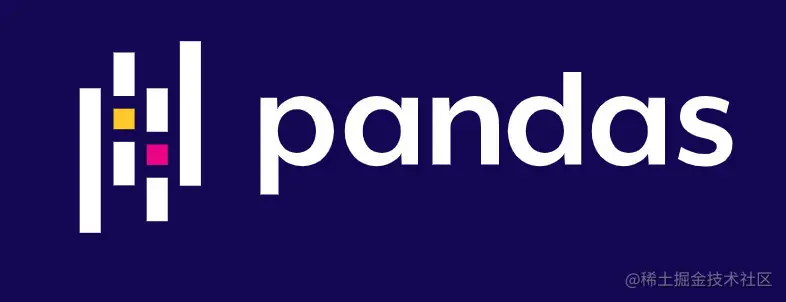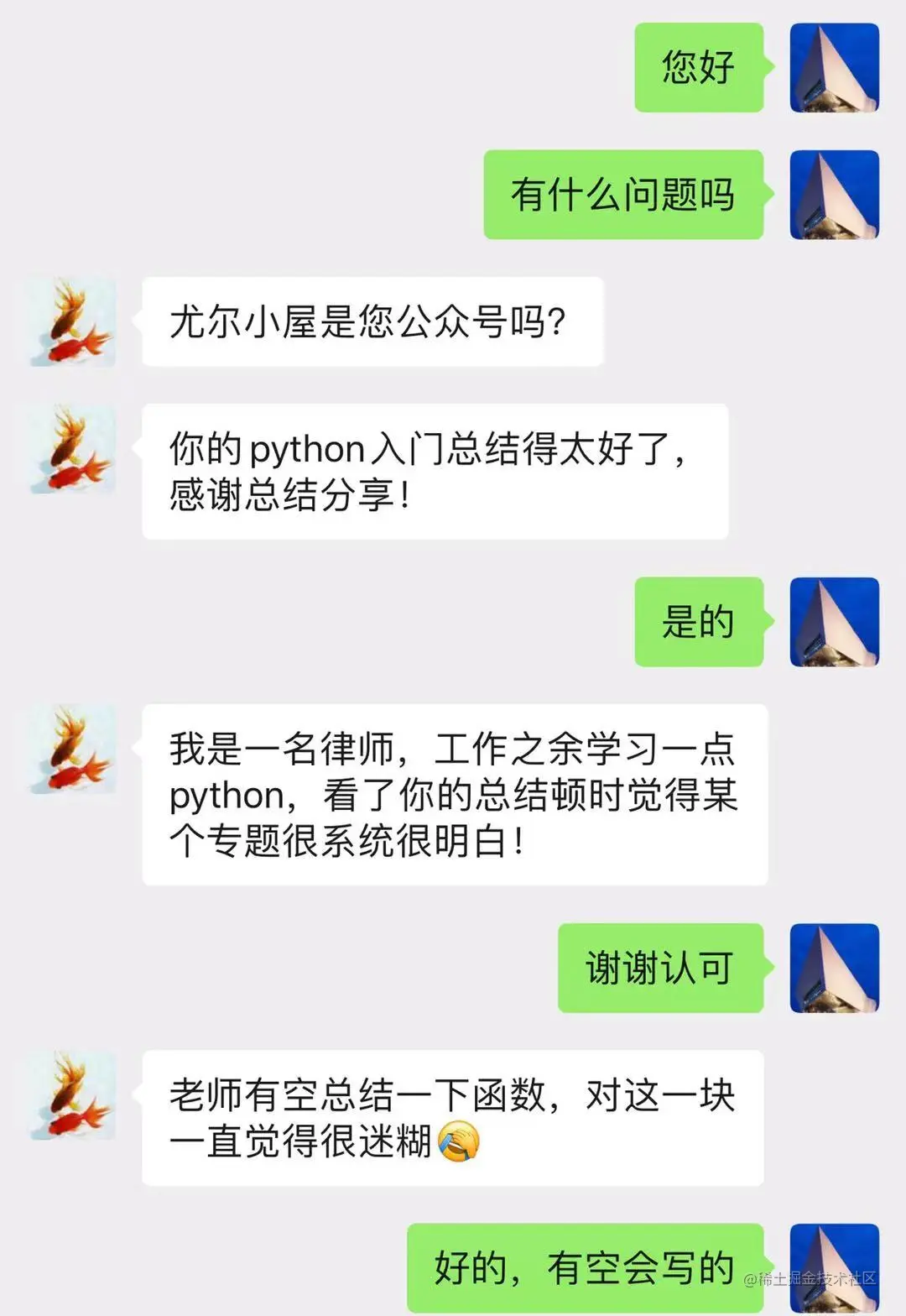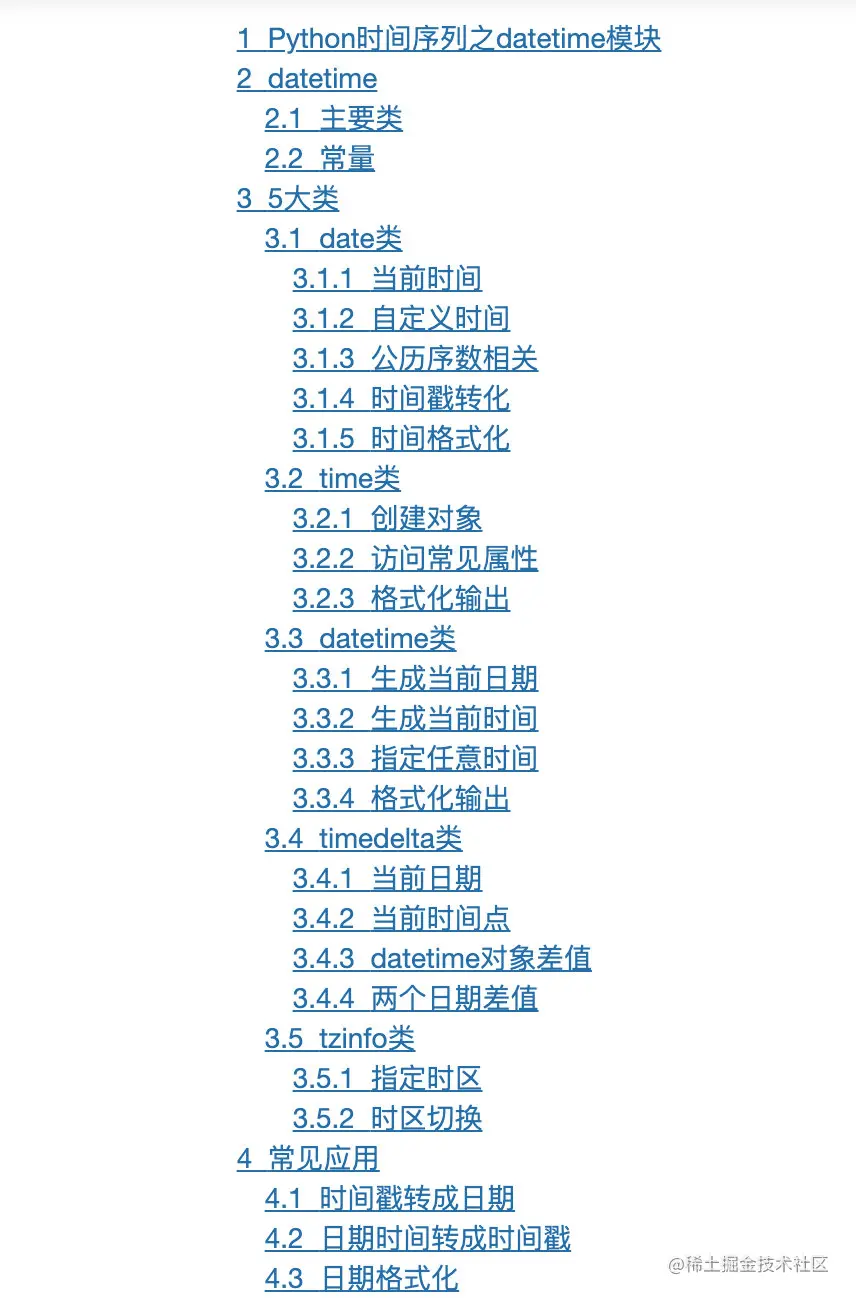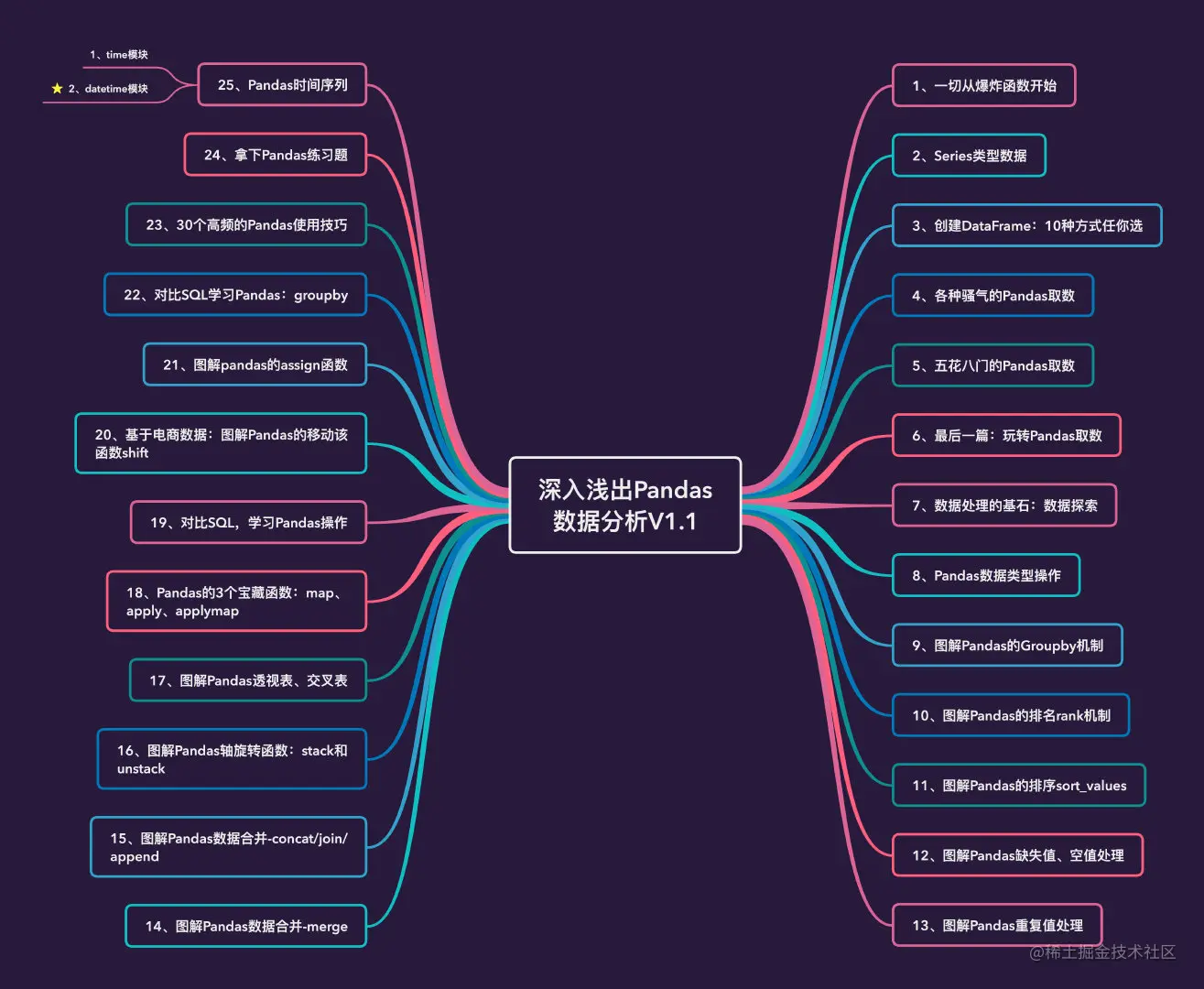# Datetime module of Python time series

official account ： Youer cottage
author ：Peter
edit ：Peter

Hello everyone , I am a Peter~

In the first chapter Python In the article of time series Peter In detail time modular , This article focuses on datetime modular .

This module can be said to be time Upgraded version of the module , Use is more common and common , The usage is also more comprehensive . The article will explain the use of this module through various examples .## gossip

Last one The lawyer By adding the official account. Peter, He studies after work Python, Is this society really so voluminous ？

friends ,Python start to learn sth. , It's best to learn from youer cabin ~## Catalog## Pandas article

Pandas Relevant articles are updated to page 26 piece , The focus in the near future is ：Python or Pandas How to deal with The time series Relevant data .

The last article was ：time Module explanation , Please refer to ：## datetime modular

### Main categories

datetime The main classes contained in the module are ：

• date： Date object , Common attributes are year, month, day etc.
• time： Time object , The main attributes are hour, minute, second, microsecond
• datetime： Date time object , attribute date And attribute datetime The combination of
• datetime_CAPI： Date object C Language interface
• timedelta： The time interval between two times
• tzinfo： Abstract base class of time zone information object

### Constant

There are mainly two constants ：

1. MAXYEAR： Returns the maximum year that can be represented ,datetime.MAXYEAR
2. MINYEAR： Returns the smallest year that can be represented ,datetime.MINYEAR

## 5 Categories:

What follows datetime Module 5 Specific use methods of large categories ：

• date
• time
• datetime
• timedelta
• tzinfo

We must import the module before we use it

``````from datetime import *   # * Represents all classes under the module
Copy code ``````

### date class

date Object by year year 、month Month and day The date consists of three parts ：

#### current time

``````#  The way 1

from datetime import date

datetime.today().date()
Copy code ``````
``````datetime.date(2021, 10, 20)
Copy code ``````
``````#  The way 2

from datetime import date

# today Is a date object , Returns the current date
today = date.today()
today
Copy code ``````
``````datetime.date(2021, 10, 20)
Copy code ``````

adopt year、month、day 3 A property descriptor to access ：

``````print(" This year, ：",today.year)   #  return today Year of object
Copy code ``````
`````` This year, ： 2021
This month, ： 10
Today, ： 20
Copy code ``````

adopt __getattribute__(...) Methods the above values were obtained ：

``````today.__getattribute__("year")
Copy code ``````
``````2021
Copy code ``````
``````today.__getattribute__("month")
Copy code ``````
``````10
Copy code ``````
``````today.__getattribute__("day")
Copy code ``````
``````20
Copy code ``````

Besides , We can also visit other date Class information ：

``````print(" The current date ：",today)  #  The current date
print(" The current date ( String form )：",today.ctime())   #  Returns a string of dates
print(" Time ( Tuple form )：",today.timetuple())   #  Time tuple information for the current date
Copy code ``````
`````` The current date ： 2021-10-20
The current date ( String form )： Wed Oct 20 00:00:00 2021
Time ( Tuple form )： time.struct_time(tm_year=2021, tm_mon=10, tm_mday=20, tm_hour=0, tm_min=0, tm_sec=0, tm_wday=2, tm_yday=293, tm_isdst=-1)
Copy code ``````
``````print(" This week ：",today.weekday())  # 0 On behalf of Monday , Reason by analogy
print(" The ordinal number of the Gregorian calendar ：",today.toordinal())  #  Returns the ordinal number of the Gregorian date
print(" year / Weeks / week ：",today.isocalendar())   #  Returns a tuple ： Weeks of the year , What day
Copy code ``````
`````` This week ： 2
The ordinal number of the Gregorian calendar ： 738083
year / Weeks / week ： (2021, 42, 3)
Copy code ``````

#### Custom time

Specify an arbitrary time ：

``````#  Customize a time

new_date = date(2021,12,8)
new_date
Copy code ``````
``````datetime.date(2021, 12, 8)
Copy code ``````
``````#  Return different properties

print("year: ", new_date.year)
print("month: ", new_date.month)
print("day: ", new_date.day)
Copy code ``````
``````year:  2021
month:  12
day:  8
Copy code ``````
``````#  Return time tuple
new_date.timetuple()
Copy code ``````
``````time.struct_time(tm_year=2021, tm_mon=12, tm_mday=8, tm_hour=0, tm_min=0, tm_sec=0, tm_wday=2, tm_yday=342, tm_isdst=-1)
Copy code ``````
``````#  Returns the Gregorian ordinal number

new_date.toordinal()
Copy code ``````
``````738132
Copy code ``````
``````#  Back to the week ,0 On behalf of the week 1,1 On behalf of the week 2

new_date.weekday()
Copy code ``````
``````2
Copy code ``````
``````#  Back to the week ,1 On behalf of the week 1,2 On behalf of the week 2

new_date.isoweekday()
Copy code ``````
``````3
Copy code ``````
``````#  Return a tuple ：（ year , Week , What day of the week ）

new_date.isocalendar()
Copy code ``````
``````(2021, 49, 3)
Copy code ``````
``````#  With ISO 8601 Format ‘YYYY-MM-DD’ return date String form of

new_date.isoformat()
Copy code ``````
``````'2021-12-08'
Copy code ``````
``````#  Returns a string of dates

new_date.ctime()
Copy code ``````
``````'Wed Dec  8 00:00:00 2021'
Copy code ``````

Specify different date output formats ：

``````#  Returns the date string in the specified format

new_date.strftime("%Y-%m-%d")
Copy code ``````
``````'2021-12-08'
Copy code ``````
``````new_date.strftime("%Y/%m/%d")
Copy code ``````
``````'2021/12/08'
Copy code ``````
``````new_date.strftime("%Y year %m month %d Japan ")
Copy code ``````
``````'2021 year 12 month 08 Japan '
Copy code ``````
``````#  Replacement time , For example, we replace new_date

r_date = new_date.replace(2021,11,10)
r_date
Copy code ``````
``````datetime.date(2021, 11, 10)
Copy code ``````

under these circumstances , We create a new date object , Of course, we can also display the specified parameters ：

``````new_date.replace(year=2021,month=11,day=11)
Copy code ``````
``````datetime.date(2021, 11, 11)
Copy code ``````

#### Gregorian ordinal number correlation

The ordinal number of the Gregorian calendar is and toordinal Method related

``````#  View the Gregorian ordinal number of the current date

to_timestamp = today.toordinal()
to_timestamp
Copy code ``````
``````738083
Copy code ``````

Convert a given Gregorian ordinal number to a specific time and date ：fromordinal

``````print(date.fromordinal(to_timestamp))
Copy code ``````
``````2021-10-20
Copy code ``````

#### Timestamp conversion

By function fromtimestamp To convert

``````import time
t = time.time()  #  Timestamp of current time
t
Copy code ``````
``````1634732660.382036
Copy code ``````
``````print(date.fromtimestamp(t))  #  Time stamp ---> date
Copy code ``````
``````2021-10-20
Copy code ``````

Convert arbitrary timestamp ：

``````date.fromtimestamp(1698382719)
Copy code ``````
``````datetime.date(2023, 10, 27)
Copy code ``````

#### Time format

``````#  Now we're right today Object to format the output

today
Copy code ``````
``````datetime.date(2021, 10, 20)
Copy code ``````
``````print(today.strftime("%Y/%m/%d"))
Copy code ``````
``````2021/10/20
Copy code ``````
``````print(today.strftime("%Y-%m-%d"))
Copy code ``````
``````2021-10-20
Copy code ``````

### time class

#### Create objects

First create an arbitrary time

``````from datetime import time

t = time(20,30,40,1000)
Copy code ``````

#### Access common properties

Hours, minutes and seconds are common attributes

``````print(t.hour)  #  when
print(t.minute) #  branch
print(t.second)  #  second
print(t.microsecond)  #  Microsecond
Copy code ``````
``````20
30
40
1000
Copy code ``````

#### Format output

``````#  return  ISO 8601 Format time string

t.isoformat()
Copy code ``````
``````'20:30:40.001000'
Copy code ``````
``````#  Specify the format of the output

t.strftime("%H:%M:%S:%f")
Copy code ``````
``````'20:30:40:001000'
Copy code ``````

alike , It also has the function of replacement ：

``````#  Implicit replacement
t.replace(14,37,8)
Copy code ``````
``````datetime.time(14, 37, 8, 1000)
Copy code ``````
``````#  Explicit substitution

t.replace(hour=4,minute=18,second=19)
Copy code ``````
``````datetime.time(4, 18, 19, 1000)
Copy code ``````

### datetime class

datetime Object contains date Objects and time All information about the object . Exclusive datetime Summary of methods and properties ：

• date(…)： return datetime The date part of the object
• time(…)： return datetime The time part of the object
• utctimetuple(…)： return UTC Time tuple part

#### Generate current date

``````from datetime import datetime

k = datetime.today()  #  Current specific time
print(k)
Copy code ``````
``````2021-10-20 20:24:23.053493
Copy code ``````

Access different attribute information of the current time ：

``````print("year:",k.year)
print("month:",k.month)
print("day:",k.day)
Copy code ``````
``````year: 2021
month: 10
day: 20
Copy code ``````

#### Generate current time

``````#  Return the current specific time

n = datetime.now()
n
Copy code ``````
``````datetime.datetime(2021, 10, 20, 20, 24, 23, 694127)
Copy code ``````
``````#  return datetime The date part of the object

n.date()
Copy code ``````
``````datetime.date(2021, 10, 20)
Copy code ``````
``````#  return datetime The time part of the object

n.time()
Copy code ``````
``````datetime.time(20, 24, 23, 694127)
Copy code ``````
``````#  return datetime Object's UTC Time tuple part

n.utctimetuple()
Copy code ``````
``````time.struct_time(tm_year=2021, tm_mon=10, tm_mday=20, tm_hour=20, tm_min=24, tm_sec=23, tm_wday=2, tm_yday=293, tm_isdst=0)
Copy code ``````

You can also generate other attribute information ：

``````#  Returns the current UTC Date and time datetime object

print(datetime.utcnow())
Copy code ``````
``````2021-10-20 12:24:24.241577
Copy code ``````
``````#  Of a given timestamp datetime object

print(datetime.fromtimestamp(1697302830))
Copy code ``````
``````2023-10-15 01:00:30
Copy code ``````
``````#  Specifies the... Of the Gregorian ordinal number datetime object

print(datetime.fromordinal(738000) )
Copy code ``````
``````2021-07-29 00:00:00
Copy code ``````
``````#  Stitching date and time

print(datetime.combine(date(2020,12,25), time(11,22,54)))
Copy code ``````
``````2020-12-25 11:22:54
Copy code ``````

#### Specify any time

``````#  Specify an arbitrary time

d = datetime(2021,9,25,11,24,23)
Copy code ``````
``````print(d.date())  #  date
print(d.time())  #  Time
print(d.timetz())  #  from datetime Split the specific time zone attribute in time

print(d.timetuple())  #  time tuples
print(d.toordinal())  #  and date.toordinal equally
print(d.weekday())  #  Two weeks
print(d.isoweekday())
print(d.isocalendar())  # ISO Format output
print(d.isoformat())
print(d.strftime("%Y-%m-%d %H:%M:%S"))  #  Specify the format
print(d.replace(year=2021,month=1))  #  Replace
Copy code ``````
``````2021-09-25
11:24:23
11:24:23
time.struct_time(tm_year=2021, tm_mon=9, tm_mday=25, tm_hour=11, tm_min=24, tm_sec=23, tm_wday=5, tm_yday=268, tm_isdst=-1)
738058
5
6
(2021, 38, 6)
2021-09-25T11:24:23
2021-09-25 11:24:23
2021-01-25 11:24:23
Copy code ``````

#### Format output

``````#  Direct formatted output of time

print(datetime.strptime("2020-12-25","%Y-%m-%d"))
Copy code ``````
``````2020-12-25 00:00:00
Copy code ``````

For given datetime Object's formatted output , For example, the instantiated object created above k：

``````k
Copy code ``````
``````datetime.datetime(2021, 10, 20, 20, 24, 23, 53493)
Copy code ``````
``````#  Format output

k.strftime("%Y-%m-%d %H:%M:%S")
Copy code ``````
``````'2021-10-20 20:24:23'
Copy code ``````

### timedelta class

timedelta Object represents a time period , Two dates (date) Date or time (datetime) Difference between .

Currently, parameters are supported :weeks、days、hours、minutes、seconds、milliseconds、microseconds.

#### The current date

``````from datetime import timedelta, date, datetime

d = date.today()  #  The current date
d
Copy code ``````
``````datetime.date(2021, 10, 20)
Copy code ``````
``````print(" today ：",d)
print(" add 3 God +8 Hours ：", d + timedelta(days=3,hours=8))  #  add 3 Days and 8 Hours
Copy code ``````
`````` today ： 2021-10-20
add 3 God +8 Hours ： 2021-10-23
Copy code ``````

#### At the current time

``````#  At the current time
now = datetime.now()
now
Copy code ``````
``````datetime.datetime(2021, 10, 20, 20, 24, 26, 777335)
Copy code ``````
``````print(now + timedelta(hours=4))  #  add 4 Hours
print(now + timedelta(weeks=2))  #  add 2 Last week
print(now - timedelta(seconds=500))  #  subtract 500 second
Copy code ``````
``````2021-10-21 00:24:26.777335
2021-11-03 20:24:26.777335
2021-10-20 20:16:06.777335
Copy code ``````

#### datetime Object difference

``````delta = datetime(2020,12,26) - datetime(2020,12,12,20,12)
print(delta)
Copy code ``````
``````13 days, 3:48:00
Copy code ``````
``````delta.days  #  Date interval ： God
Copy code ``````
``````13
Copy code ``````
``````delta.seconds  #  Date interval ： second
Copy code ``````
``````13680
Copy code ``````
``````delta.total_seconds()  # #  Turn it all into seconds
Copy code ``````
``````1136880.0
Copy code ``````

#### The difference between the two dates

``````d1 = datetime(2021,10,1)
d2 = datetime(2021,10,8)
Copy code ``````
``````d1.__sub__(d2)  # d1 - d2
Copy code ``````
``````datetime.timedelta(days=-7)
Copy code ``````
``````d2.__sub__(d1) # d2 - d1
Copy code ``````
``````datetime.timedelta(days=7)
Copy code ``````
``````# rsub To express with d2 - d1

d1.__rsub__(d2)
Copy code ``````
``````datetime.timedelta(days=7)
Copy code ``````

The difference between the above two dates is datetime.timedelta, If you get an integer type result, do the following ：

``````d1.__sub__(d2).days
Copy code ``````
``````-7
Copy code ``````

### tzinfo class

The main function is to specify the time zone of the time

#### Designated time zone

``````from datetime import date, timedelta, datetime, timezone

tz_utc_8 = timezone(timedelta(hours=8)) #  Create a time zone
print(tz_utc_8)
Copy code ``````
``````UTC+08:00
Copy code ``````
``````now = datetime.now()
print(now)
Copy code ``````
``````2021-10-20 20:24:28.844732
Copy code ``````
``````new_time = now.replace(tzinfo=tz_utc_8)  #  Force to add 8 Hours
print(new_time)
Copy code ``````
``````2021-10-20 20:24:28.844732+08:00
Copy code ``````

#### Time zone switching

``````#  obtain UTC Time
utc_now = datetime.utcnow().replace(tzinfo=timezone.utc)  #  Appoint utc The time zone
print(utc_now)
Copy code ``````
``````2021-10-20 12:24:29.336367+00:00
Copy code ``````
``````#  adopt astimezone Switch to East eight

beijing = utc_now.astimezone(timezone(timedelta(hours=8)))
print(beijing)
Copy code ``````
``````2021-10-20 20:24:29.336367+08:00
Copy code ``````
``````# UTC Time zone switch to East nine ： Tokyo time

tokyo = utc_now.astimezone(timezone(timedelta(hours=9)))
print(tokyo)
Copy code ``````
``````2021-10-20 21:24:29.336367+09:00
Copy code ``````
``````#  Beijing time. （ East eight ） Switch directly to Tokyo time （ East nine ）

tokyo_new = beijing.astimezone(timezone(timedelta(hours=9)))
print(tokyo_new)
Copy code ``````
``````2021-10-20 21:24:29.336367+09:00
Copy code ``````

## Common applications

### Time stamp to date

``````import time
now_timestamp = time.time()
now_timestamp
Copy code ``````
``````1634732670.286224
Copy code ``````
``````# 1- Turn to a specific point in time

now = time.ctime(now_timestamp)
print(now)
Copy code ``````
``````Wed Oct 20 20:24:30 2021
Copy code ``````
``````# 2- The timestamp is first converted into a time tuple ,strftime After converting to the specified format

now_tuple = time.localtime(now_timestamp)
print(now_tuple)
Copy code ``````
``````time.struct_time(tm_year=2021, tm_mon=10, tm_mday=20, tm_hour=20, tm_min=24, tm_sec=30, tm_wday=2, tm_yday=293, tm_isdst=0)
Copy code ``````
``````time.strftime("%Y/%m/%d %H:%M:%S", now_tuple)
Copy code ``````
``````'2021/10/20 20:24:30'
Copy code ``````
``````#  Select a specific timestamp

timestamp = 1618852721

a = time.localtime(timestamp)  #  Get data in time tuple form
print(" Time tuple data ：",a)
time.strftime("%Y/%m/%d %H:%M:%S", a)  #  format
Copy code ``````
`````` Time tuple data ： time.struct_time(tm_year=2021, tm_mon=4, tm_mday=20, tm_hour=1, tm_min=18, tm_sec=41, tm_wday=1, tm_yday=110, tm_isdst=0)

'2021/04/20 01:18:41'
Copy code ``````
``````time.ctime(1618852741)
Copy code ``````
``````'Tue Apr 20 01:19:01 2021'
Copy code ``````

### Date time to timestamp

Given a string type of date data , How to convert it into the time format we want ？

``````date = "2021-10-26 11:45:34"

# 1、 Time string into time array form
date_array = time.strptime(date, "%Y-%m-%d %H:%M:%S")
date_array
Copy code ``````
``````time.struct_time(tm_year=2021, tm_mon=10, tm_mday=26, tm_hour=11, tm_min=45, tm_sec=34, tm_wday=1, tm_yday=299, tm_isdst=-1)
Copy code ``````
``````# 2、 Look at the time array data

print(" Time array ：", date_array)
Copy code ``````
`````` Time array ： time.struct_time(tm_year=2021, tm_mon=10, tm_mday=26, tm_hour=11, tm_min=45, tm_sec=34, tm_wday=1, tm_yday=299, tm_isdst=-1)
Copy code ``````
``````# 3、mktime Time array into time stamp

time.mktime(date_array)
Copy code ``````
``````1635219934.0
Copy code ``````

### Date formatting

``````import time

old = "2021-09-12 12:28:45"

# 1、 Convert to a time array
time_array = time.strptime(old, "%Y-%m-%d %H:%M:%S")

# 2、 Convert to a new time format (2021/09/12 12-28-45)
new = time.strftime("%Y/%m/%d %H-%M-%S",time_array)  #  Specify the display format

print(" Original format time ：",old)
print(" New format time ：",new)
Copy code ``````
`````` Original format time ： 2021-09-12 12:28:45
New format time ： 2021/09/12 12-28-45
Copy code ``````